An Overview of Geometries
[a work in progress, it's completed but has yet to be entirely transcribed into HTML]

### 1) Algebraic

Algebraic geometry is a branch of mathematics in which the properties of a geometrical structure are described by means of algebraic expressions.

Consider:

Coordinate Axes 'X' and 'Y' ('S' will stand for the usual summation symbol):

The relation between 'x' and 'y' coordinates (x0,..., xn) and (y0,..., yn) of a specific point is given by the linear equations:

 py i = S ai jxj(for j=0 to n) lxi = S bi jyj(for j=0 to n) ai j, bi j : coefficients p, l : proportionality factors
*COMMENT*
*Algebraic Geometry looks very much like the Theory of Polynomials in Abstract Algebra and Algebraic Topology.*

Given an allowable coordinate system in Pn, a projective space, a set of homogeneous polynomial equations such as:

fi (x0,..., xn) = 0

are derived in which the coefficients are constants. The aggregate of all points such as: (a0,..., an) satisfying the polynomial equations form what is known as an algebraic Variety in Pn. This definition does not depend on the coordinate system chosen.

Modern algebraic geometry is mainly concerned with properties of Varieties that are independent of the projective spaces in which the Varieties are embedded.

Much of classical algebraic geometry is devoted to the study of properties of Varieties invariant under bi-rational (U' Í U, V' Í V, U' <---> V') transformations.

A) The study of algebraic varieties is facillitated by means of local rings.

B) Irreducible subvarities of dimension zero <---> point.

C) A subvariety is considered a 'set'.

Considering A, B, and C, (from above) a variety 'U' is then a space 'X' consisting of points endowed with the Zarisky Topology with a ring associated with each 'point.' The set of rings is said to form a 'sheaf' over 'X'.

An algebraic variety can be represented as a ringed space.

The definition of sheaves can be extended to define sheaves of groups or other sets with an algebraic structure.

*The gist seems to be to regard algebraic varieties as ringed spaces and then studying the properties of sheaves over them.*

An affine variety considered as a ringed space is defined by an integral domain R(V, k), and the 'points' aredefined by the maximal prime ideals of R(V, k), where 'V' is the Variety and 'k' is the field. A polynomial 'vanishing' on a variety is a property of an ideal.

A sequence of sheaves and homomorphisms is referred to as 'exact' if the image of each homomorphism is the kernel of the following one [---> F1 ---> F2 ---> F3 --->....].
*Sounds like Chain Maps from Homology Theory.*

Other concepts:

pre-sheaf,
schema,
pre-schema,
coherent,
morphism,
stalk,
genus

A coherent sheaf over P (projective space) is just a finite-dimensional vector space over k (a field).

BOOKS:

"Foundations of Algebraic Geometry" by Andre Weil; and,
"Introduction to Algebraic Geometry" by Serge Lang.

### 2) Analytic

The use of algebra to:

1) manipulate the dimensions of geometric figures;
2) the representations of a point by a pair of numbers; and,
3) the representation of lines and curves by equations.

The basis of analytic geometry is the idea that a point in space can be specified by numbers giving its position.

General equations for a line:

ax + by + c =0;
y = mx + d (the y intercept form);
x/x0 + y/y0 = 1 (x0 , y0), known point on line

Also:

a ratio of differences:
(y - y1)/(y2 - y1) = (x - x1)/(x2 - x1)
(a line through (x1 , y1) and (x2 , y2)

#### Determinants

 | x y 1|| x1 y1 1| = 0| x2 y2 1|2 points | x1 y1 1|| x2 y2 1| = 0| x3 y3 1|3 points

## Conic Sections

A circle is a special case of an ellipse.

General Equation:
Ax² + Bx²y + Cxy² = Dx + Ey +F = 0

Ellipse:
x²/a² + y²/b² = 1

eccentricity < 1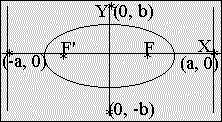Hyperbola:
x²/a² - y²/b² = 1

eccentricity > 1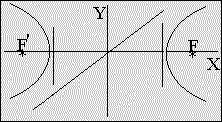Parabola:
y² = 2 · px

eccentricity = 1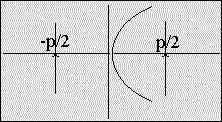*The parabola may be though of as a limiting case between an ellipse (e < 1) and a hyperbola (e > 1). It also may be considered as an elongated ellipse with its center, one focus and vertex all coinciding at infinity. The graph of a quadratic polynomial, y = ax² + bx + c, is also a parabola.*

Ax² + Bxy + Cy² + Dx + Ey + F = 0
(general equation for conic sections)

#### Cases for (B² - 4 · A · C) defined as the eccentricity:

1) < 1: an ellipse
2) > 1: a hyperbola
3) = 1: a parabola

The coefficients, A, B, C, D, E, and F are completely determined by the eccentricity and the position(s) of the focus(foci) and directrix.

#### Cases for (B² - 4 · A · C) and the determinant (det) of coefficients:

1) (B² - 4 · A · C) = 0
det not equal to 0: a parabola
det = 0: 2 parallel or coincident lines, or, there is no curve.

2) (B² - 4 · A · C) < 0

det not equal to 0: a hyperbola
det = 0: 2 intersecting lines

3) (B² - 4 · A · C) > 0

det not equal to 0: an ellipse
det = 0: single point

### 3) Trigonometry

Trigonometry is the branch of mathematics concerned with specific functions of angles and their applications to calculations in geometry. The trigonometric functions are considered properties of the angle in question. Trigonometry unites aspects of arithmetic, algebra and geometry.

The 'radian' is the angle at the center of a circle that intercepts an arc equal in length to the radius:

#### 1 revolution = 4 right angles = 360° = 2Pi radians

In application the essential problem is the solution of triangles.

The fundamental configuration to which the formulas of Spherical Trigonometry apply is the trihedral angle formed by 3 rays radiating from a point.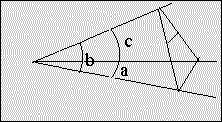COS a = COS b · COS c + SIN b · SIN c · COS a

Area of a Spherical Triangle: A = E · r ²

r is the radius of the sphere, and E is the excess of the sum of the 3 dihedral angles (opposite to the face angles) over 2 right angles measured in radians.

### 3a) Analytic Trigonometry

Trigonometric functions of a real variable 'x' are defined by means of a trigonometric functions of an angle in terms of radians. The period for sin, cos, sec, and cosec is 360° equal to 2Pi radians; Pi for tan and cot.

Polar Coordinates: (r, O)
Linear Transformation:
x = r · cos(O), y = r · sin(O)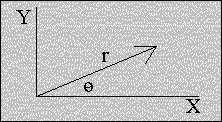The polar equation of a circle expresses the fact that
the curve is independent of O and has constant radius.

Translation:
x = x' + h, y = y' + h

*A combination of dimensions describing a given system forces the centering of the highest degree components in terms of the whole system.*

Rotation:
x = x' · cos(O) - y · sin(O)
y = x' · sin(O) + y' · cos(O)

The Discriminant and the expression (B² - 4 · A · C), from the general equation for a conic, are invariants for rotational and translational transformations.

If 3 masses, t1, t2, and t3 are placed at points P1, P2 and P3 of the Euclidean plane, their total mass appears to act at point P on the plane P1P2P3 (triangle of reference). These are called the Barycentric coordinates of the point P (centroid, center of mass).

*They are homogeneous coordinates because for any 'k' not equal to 0, masses, kt 1, kt 2, and kt 3 have the same centroid as t 1, t 2 and t 3. These functions are rational.*

The extension of the Euclidean plane by postulating a line at infinity, the points of which lie on parallel lines called 'pencils', is a projective plane. In terms of Barycentric coordinates, the equation of the line at infinity restricts the {t k} to be: t 1 + t 2 + t 3 = 0.

General projective coordinates (x 1, x 2, x 3) are derived by the substitution t i = k i x i in which the coefficients k i are constants. The line at infinity can now be expresed as a linear combination of coordinates, k 1 x 1 + k 2 x 2 + k 3 x 3 = 0, and any other homogeneous linear equation represents an ordinary line. The transition to projective geometry is completed by waiving the distinction, so that a point at infinity is treated just like any other point. It enables the points of the projective plane to be defined as the ordered triads of numbers (x 1, x 2, x 3), not all zero, with the convention that (kx 1, kx 2, kx 3) is the same point for all 'k' not equal to zero.

The general homogeneous linear transformation: x i = S j c i j x j, in which the determinant (cij) is not equal to zero, transforms collinear points into collinear points, a collineation. For example: the collineation that leaves unchanged the first two coordinates and modifies the third with multiplication by a constant:

x'1 = x 1, y' 1 = y 1, z' 1 = kz 1

or, in this case:

x'1 = x1, x'2 = x2, x'3 = x3,

is a homotopy that leaves invariant every line through the point (0, 0, 1) and every point on the line x3 = 0.

#### Coordinates:

1) Cartesian
2) Polar
a) cylindrical (r, O, z), in which 'r' and 'O' are related to 'x' and 'y' in the usual way,
b) spherical, which consist of distance from origin, latitude and longitude

3) Barycentric and all other projective coordinates are referred to a tetrahedron P1P2P3P4

#### Special Curves

1. Cubical Parabola:
y = ax3 + bx2 + cx + d
or,
y = ax2 (x - e)
2. Semi-cubical Parabola:
x3 = Ay2
The curve along which a particle may descend under the action of gravity so as to describe vertical spaces in equal times.
3. Sinusoidal Spirals:
rn = an · cos(nO)
for:
n = -1, a line
n = +1, a circle
n = 1/2, a cardiod
n = -1/2, a parabola
n = -2, an equalateral triangle
n = +2, a lemniscate

4. A Cycloid is the locus of a point on the circumference of a circle of radius 'r' that rolls without slipping along a straight line: x = r(O - sinO), y = r(1 - cosO). A Cycloid is a kind of Brachistochrone (no, it's not a dinosaur). It is a curve along which a body moves from one point to another under the action of an accelerating force in the least time possible.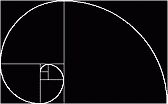5. A Logarithmic spiral is the curve cutting radius vectors from a fixed point 'x' under a constant angle '*'. The most famous example of which is the "Golden Spiral."
r = ke c#
c = cot(*)
The form depends on 'c' independent of 'k.' The pole 'x' is an asymptotic point. The pedal of a logarithmic spiral with respect to its pole is a logarithmic spiral, and its evolute is an equal spiral with the same asymptotic point.
6. A Catenary is the form that a chain assumes when suspended by its ends and acted upon by gravity alone. Euler discovered, in 1744, that a catenary revolved about its asymptote generates a catenoid, the only minimal surface of revolution. The evolute of the tractrix is a catenary. The tractrix revolved about it asymptote generates a pseudosphere, a surface of constant negative curvature.
7. A Caustic is an envelope of rays emanating from a source.
8. An Evolute is the envelope of a given curve or, what amounts to the same thing, the locus of the centres of curvature of a given curve. There are an infinite number of such trajectories to a givencurve, and they are said to be parallel curves, i.e., any two cut off equal lengths on common normals.
9. Isotopic curve: the locus of points of intersection of tangents to a given curve (or a pair of curves) meeting at a constant angle. For a right angle, the isotopic curve is an orthoptic curve. The orthoptic of a parabola is its directrix, of a central conic, its concentric circle; and of a deltoid, a circle.
10. A Roulette is the envelope of a fixed line situated in the plane of a curve that rolls without slipping on a fixed curve or straight line.
11. A Tautochrone is a curve down which a particle acted upon by specified forces will descend in the same amount of time from any initial point to the lowest point. When gravity is the force considered, the inverted cycloid with axis vertical has the required property.
12. A Loxodrome or spherical helix is defined as the curve cutting the meridian of a sphere at a constant angle.
13. A Helix is the curve cutting the generators of a right circular cylinder under a constant angle. The orthogonal projection of a helix on a plane parallel to the axis of the cylinder is a sine curve.
14. A skewed or twisted cubic is the locus of the common point of three projective axial pencils of planes.

### 4) Differential

Differential geometry has its origin in the discovery of the infinitesimal calculus, one part of mathematics that deals with limits.

#### Equations of a Surface:

1. xi = xi (U1, U2) for i = 1, 2, 3
(U1, U2) is a point on the surface.
2. Vector component form: Ei (U1, U2) for i = 1, 2, 3

The geometric properties of a surface are then completely determined by two quadratic differential forms:
1. ds2 = Si dx2i
2. - Si dxi · dEi for i = 1, 2, 3

Riemann Geometry: the geometry in a space of n-dimensions with the coordinates ua, a = 1,...,n, based on a quadratic differential form:

Sa,b ga,b (u) dua · dub, for a,b = 1,...,n.

This geometry is of general form and includes as special cases the non-Euclidean geometries. It serves as a model of the physical Universe in Einstein's General Theory of Relativity.

Modern differential geometry stems from the basis that the objects of study are a class of spaces called *manifolds* equipped with additional structures. The main problems deal with the global properties that arise only when the manifolds are looked on as a whole. In global differential geometry, topology is a major tool.

A manifold is a space that is covered by a finite or a countable number of coordinate charts with each point in a chart described by the real coordinates x 1, ...,x n (called local coordinates), and such that when a point belongs to two charts and has two sets of local coordinates they are related by a transformation:

The Jacobian:

J = d(f 1,...f n)/d(x 1,...x n)

for:

{x i, x i j i,j = 1...n
{x 'i = f i (x 1,...,x n)

The determinant is non-singular, composed of continuous first partial derivatives (orthogonal). If a relation, F (f 1...f n) = 0 exists between the dependent variables, then J(f i/x i) = 0 and conversley, if the Jacobian equals zero, the f i's are related by at least one equation.

If all such functions f i have continuous partial derivatives of orders equal to or less than k, the manifold is said to be of class k. If all such functions have continuous partial derivatives of all orders, the manifold is said to be of class infinite. The local coordinates themselves have no geometric meaning, and the analytic tools for the study of manifold must be furnished by concepts that behave in a simple way under a transformation.

A tensor of contravariant order r and covariant order s is defined by specifiying its components in each coordinate chart. The tensor is called contravariant if s = 0, and covariant if r = 0.

A tensor of order one reduces to a vector. The vanishing of a tensor (i.e., of all components) is a condition invariant with a change of local coordinates.

All the tensors of given orders at a point form a vector space and the collection of all of them forms a tensor bundle of the manifold M.

All the contravariant vectors form a tangent bundle of M; covariant, cotangent.

The tensor bundles are special cases of a general vector bundle over M. A vector bundle over M is a collection of vector spaces over the points of M such that it is locally a product and that the linear structures on the vector spaces (fibres) have a respective representation.

Together with "virtual bundles" (negative numbers in arithmetic) all the finite linear combinations of the vector bundles over M are made into a group. Through the tensor product of vector bundles the group acquires a multiplication and has a ring structure called the Grothendrisk Ring, a significant global invariant over M.

The space of all frames at a point P of M is a fibre and is called the principal fibre space; its dimension is n 2 + n.

A real-valued function is a tensor field of order zero, and its partial derivatives are the components of a covariant vector field called the gradient.

Exterior differential forms are the integrals of multiple integrals.

If D is the boundary of an (r+1) dimensional domain F, then the integral of w (an arbitrary quantity) over domain D is equal to the integral of dw over domain F.

Another important operation for tensor fields is the Lie derivative:

• X, contravariant vector field
• A k, components (k = 1,...,n)
• x i, local coordinates (i = 1,...,n)
• f, real-vaued functions

X f = S A k (d/d(x k) f

is the directional derivative of f relative to X. Thus X is a linear differential operator on functions.

A vector field X defines an infinitesimal transformation which leads to a new tensor field of the same type:

d x i = A i dt, i = 1,...,n

Generally, this does not occur, however, because the local coordinates have no geometrical meaning. A structure on the manifold that makes this possible is called an affine connection. It is given by a set of components in each chart.

A Riemannian manifold has a uniquely determined connection, the Levi-Civita connection which is characterized by:

• the length of a vector remains unchanged under parallelism; and
• T ji k = T jk i (symmetry of connection)
(T ji k, set of components in each chart)

In the special case of the affine space where the connection is defined by T ji k = 0, parallelism of A j k means the constancy of all the components.

An exterior differntial form is called closed if its extrerior derivative is zero; it's called a derived form if it is the exterior derivative of another.

Two closed forms belong to the same class iff (if and only if) their difference is a derived form.

*A manifold is compact if it can be covered by a finite number of coordinate charts.

All the classes of closed forms of degree # form a real vector space. This space is manageable in the sense that contrast exists to the space of all forms of degree # or even that of all closed forms, both of which are infinitely dimensional.

The dimension of a compact manifold represented by the above described space is the #th Betti number of the manifold. The Betti number is an integer and is a topological invariant of the manifold that describes its connective properties.

Aside: What does closure have to do with homogeneity?

A form is called harmonic if it is both closed and co-closed.

On a compact orientable Riemannian manifold there is exactly one harmonic form in every class of closed forms of degree #.

If an exterior differential form is independent of the choice of the local chart, then the group of form classes are defined over the whole manifold, and are closed.

Pontryagin Characteristic (k, even):
A fundamental theorem on characteristic classes postulates that for odd k, Pk is a derived form, whereas for even k, Pk determines a class.

Top Of Page